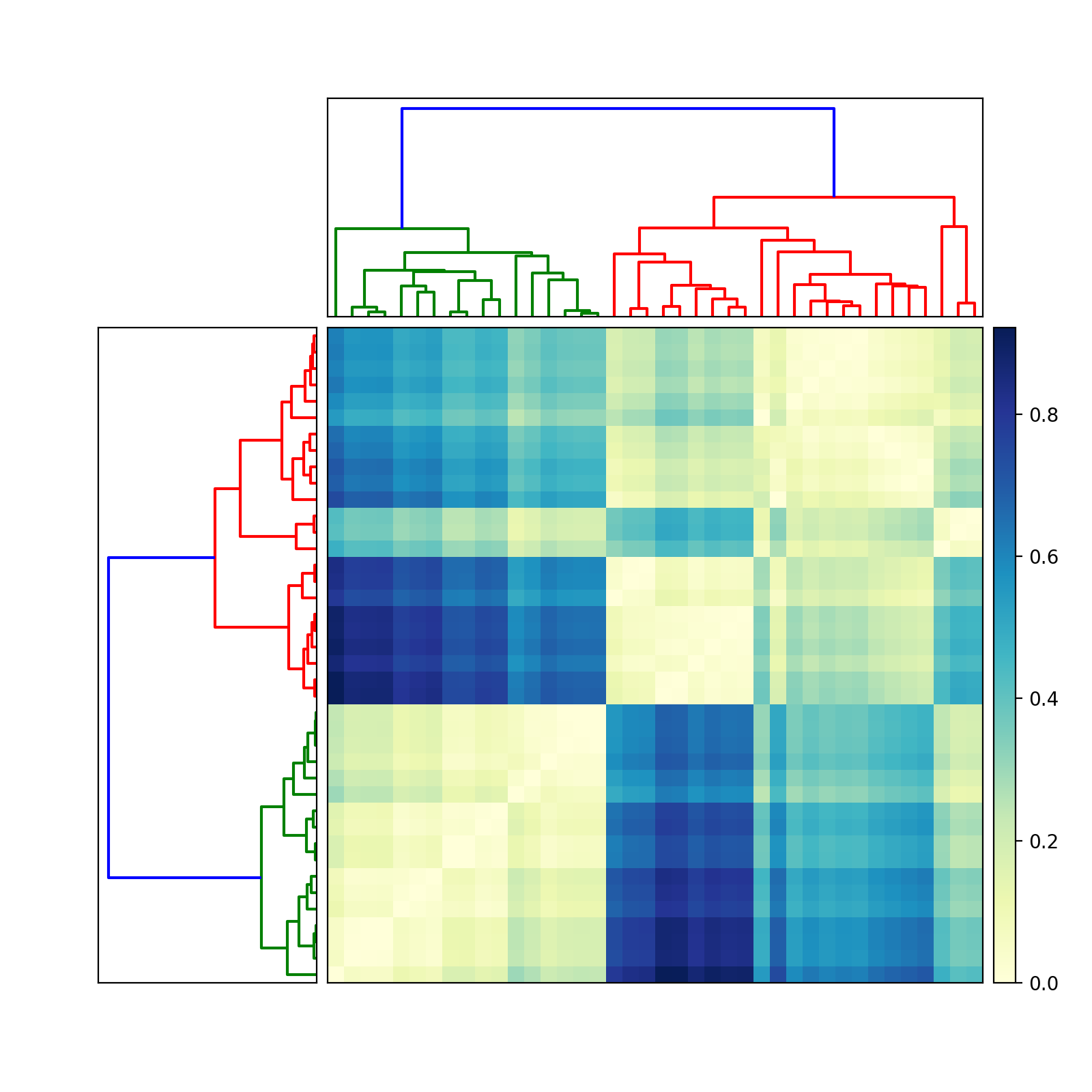2 views

How can I plot a dendrogram right on top of a matrix of values, reordered appropriately to reflect the clustering, in Python?

I use scipy.cluster.dendrogram to make my dendrogram and perform hierarchical clustering on a matrix of data. How can I then plot the data as a matrix where the rows have been reordered to reflect a clustering induced by the cutting the dendrogram at a particular threshold, and have the dendrogram plotted alongside the matrix? I know how to plot the dendrogram in scipy, but not how to plot the intensity matrix of data with the right scale bar next to it.

Any help on this would be greatly appreciated.

by (33.1k points)

A Dendrogram is a type of Hierarchical clustering that illustrates the arrangement of the clusters produced by the corresponding analyses.

Code for Dendrogram implementation using SciPy:

import scipy

import pylab

import scipy.cluster.hierarchy as sch

from scipy.spatial.distance import squareform

# Generate random features and distance matrix.

x = scipy.rand(40)

D = scipy.zeros([40,40])

for i in range(40):

for j in range(40):

D[i,j] = abs(x[i] - x[j])

condensedD = squareform(D)

# Compute and plot first dendrogram

fig = pylab.figure(figsize=(8,8))

Z1 = sch.dendrogram(Y, orientation='left')

ax1.set_xticks([])

ax1.set_yticks([])

# Compute and plot second dendrogram.

Z2 = sch.dendrogram(Y)

ax2.set_xticks([])

ax2.set_yticks([])

# Plot distance matrix.

idx1 = Z1['leaves']

idx2 = Z2['leaves']

D = D[idx1,:]

D = D[:,idx2]

im = axmatrix.matshow(D, aspect='auto', origin='lower', cmap=pylab.cm.YlGnBu)

axmatrix.set_xticks([])

axmatrix.set_yticks([])

# Plot colorbar.

pylab.colorbar(im, cax=axcolor)

fig.show()

fig.savefig('dendrogram.png')

Output: